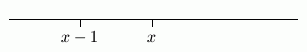﻿ Identifying Inequalities with Variables as True or False
IDENTIFYING INEQUALITIES WITH VARIABLES AS TRUE OR FALSE
• PRACTICE (online exercises and printable worksheets)
Want more details, more exercises? Read the full text!

An Inequality that is Always True

Consider the inequality ‘$\,x < x + 1\,$’.
Let $\,x\,$ be any real number.
Then, $\,x+1\,$ lies one unit to the right of $\,x\,$ on a number line.
Therefore, $\,x\,$ always lies to the left of $\,x+1\,$,
so the sentence ‘$\,x < x + 1\,$’ is always true.An Inequality that is Always False

Consider the inequality ‘$\,x -1> x\,$’.
Let $\,x\,$ be any real number.
Then, $\,x-1\,$ lies one unit to the left of $\,x\,$ on a number line.
Therefore, $\,x-1\,$ never lies to the right of $\,x\,$,
so the sentence ‘$\,x-1 > x\,$’ is always false.For these exercises, you should think in terms of position on a number line,
as in the previous examples.

EXAMPLES:

Determine if each inequality is ALWAYS TRUE or ALWAYS FALSE.

‘$\,x-1 < x + 1\,$’     is     Always True
‘$\,x > x + 2\,$’     is     Always False
Master the ideas from this section
Solving Equations of the Form $\,xy = 0$